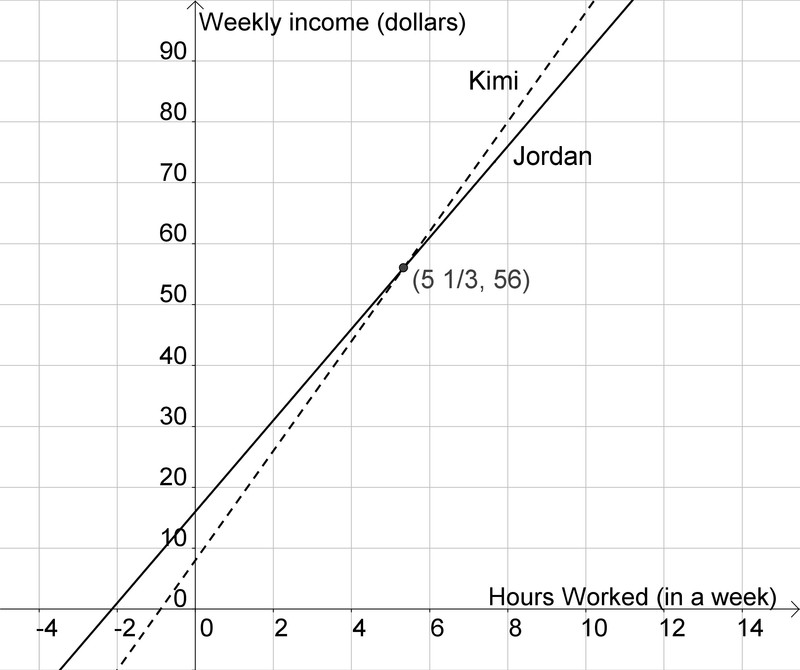# Kimi and Jordan

Alignments to Content Standards: 8.EE.C.8

Kimi and Jordan are each working during the summer to earn money in addition to their weekly allowance, and they are saving all their money. Kimi earns \$9 an hour at her job, and her allowance is \$8 per week. Jordan earns \$7.50 an hour, and his allowance is \$16 per week.

1. Complete the two tables shown below.

 Number of hours worked in a week, $h$ 0 1 2 3 4 5 6 7 Kimi's weekly total savings, $K$
 Number of hours worked in a week, $h$ 0 1 2 3 4 5 6 7 Jordan's weekly total savings, $J$

2. Write an equation that can be used to calculate the total of Kimi's allowance and job earnings at the end of one week given the number of hours she works.
3. Write an equation that can be used to calculate the total of Jordan's allowance and job earnings at the end of one week given the number of hours worked.
4. Sketch the graphs of your two equations on one pair of axes.
5. Jordan wonders who will save more money in a week if they both work the same number of hours. Write an answer for him.

## IM Commentary

The purpose of the table in (a) is to help students complete (b) by noticing regularity in the repeated reasoning required to complete the table (Standard for Mathematical Practice 8).

## Solutions

Solution: 1

1.  Number of hours worked in a week, $h$ 0 1 2 3 4 5 6 7 Kimi's weekly total earnings, $K$ 8 17 26 35 44 53 62 71
 Number of hours worked in a week, $h$ 0 1 2 3 4 5 6 7 Jordan's weekly total earnings, $J$ 16 23.5 31 38.5 46 53.5 61 68.5
2. Kimi's total savings, $K$, is the sum of her \$8 dollar allowance and (\$9 per hour $\times$ the number of hours she works, $h$). In other words, $K = 8+9h$.

3. Similarly, Jordan's total savings, $J$, is given by $J = 16+7.5h$.
4.5. The two graphs are shown above.

6. We can see from the graph that if Kimi and Jordan only work a few hours per week, Jordan will save more money. But at some point between 4 and 6 hours of work per week, Kimi's savings overtake Jordan's savings and from that point on, she will save more money. We can find out for what number of hours of work Kimi and Jordan will make the same amount of money. We do this by setting the expressions for Kimi's total and Jordan's total equal to each other, $9h+8=7.5h+16$, and solving for $h$. This yields $h=5\frac13$. This tells us that if they both work the same number of hours per week, Jordan will make more money than Kimi if they work less than $5\frac13$ hours. If, however, they work more than $5\frac13$ hours, then Kimi will make more money.

Solution: Alternate (e) solution

e. Kimi's allowance is \$8 less than Jordan's, but her hourly rate is \$1.50 higher. So for only a few hours worked, she will earn less per week than Jordan, but if she works a lot of hours, her higher hourly rate will make up for the lower allowance. We can find the number of hours at which this change occurs by calculating how many hours, at $\$1.50$more per hour, it will take to make$\$8$: $$\frac{\8}{\frac{\1.50}{\text{ hour}}} = 5\frac13 \text{ hours},$$ or $5$ hours and $20$ minutes.